Next: Computational Demonstrations: The Two-link Up: Illustration: the Event-learning Algorithm Previous: Formalizing Event-Learning in the

## Event-Learning with the SDS Controller

In this subsection we review a particular controller for continuous dynamical systems, the static and dynamic state (SDS) feedback controller proposed by Lorincz and colleagues [Szepesvári et al.(1997),Szepesvári and Lorincz(1997)], for more details see the Appendix. It is shown that it can be easily inserted into the E-learning scheme.

The SDS control scheme gives a solution to the control problem called speed field tracking5 (SFT) in continuous dynamical systems [Hwang and Ahuja(1992),Fomin et al.(1997,Szepesvári and Lorincz(1998)]. The problem is the following. Assume that a state space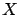and a velocity field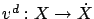are given. At time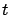, the system is in state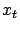with velocity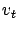. We are looking for a control action that modifies the actual velocity to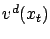. The obvious solution is to apply an inverse dynamics, i.e., to apply the control signal in state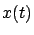which drives the system into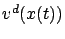with maximum probability: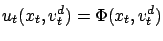Of course, the inverse dynamics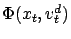has to be determined some way, for example by exploring the state space first.

The SDS controller provides an approximate solution such that the tracking error, i.e.,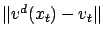is bounded, and this bound can be made arbitrarily small. This represents considerable advantage over approximations of the inverse dynamics, which can be unbounded and therefore may lead to instabilities when used in E-learning.

Studies on SDS showed that it is robust, i.e., capable of solving the SFT problem with a bounded, prescribed tracking error [Fomin et al.(1997),Szepesvári et al.(1997),Szepesvári and Lorincz(1997),Szepesvári(1998)]. Moreover, it has been shown to be robust also against perturbation of the dynamics of the system and discretization of the state space [Lorincz et al.(2002)]. The SDS controller fits real physical problems well, where the variance of the velocity field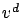is moderate.

The SDS controller applies an approximate inverse dynamics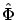, which is then corrected by a feedback term (for the sake of convenience, we use the shorthand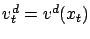). The output of the SDS controller is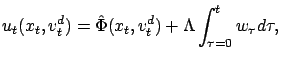where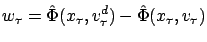is the correction term, and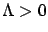is the gain of the feedback. It was shown that under appropriate conditions, the eventual tracking error of the controller is bounded by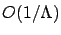. The assumptions on the approximate inverse dynamics are quite mild: only sign-properness is required [Szepesvári et al.(1997),Szepesvári and Lorincz(1997)].6Generally, such an approximate inverse dynamics is easy to construct either by explicit formulae or by observing the dynamics of system during learning.

The above described controller cannot be applied directly to E-learning, because continuous time and state descriptions are used. Therefore we have to discretize the state space, and this discretization should satisfy the condition of `sign-properness'. Furthermore, we assume that the dynamics of the system is such that for sufficiently small time steps all conditions of the SDS controller are satisfied.7 Note that if time is discrete, then prescribing desired velocityis equivalent to prescribing a desired successor state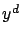[Lorincz et al.(2002)]. Therefore the controller takes the form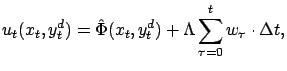where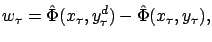and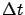denotes the size of the time steps. Note that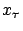and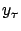(therefore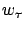) change at discretization boundaries only, i.e., when an event is observed. Therefore, event-learning with the SDS controller has more relaxed conditions on update rate than other reinforcement learning methods [Lorincz et al.(2002)].

The above defined controller can be directly inserted into event-learning by setting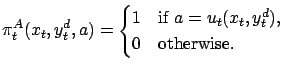(10)

Note that the action space is still infinite.

Corollary 4.3   Assume that the environment is such that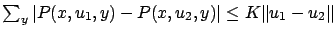for all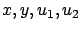.8 Let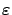be a prescribed number. For sufficiently large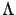and sufficiently small time steps, the SDS controller described in Equation 10 and the environment form an-MDP.

The proof can be found in Appendix D.

Consequently, Theorem 3.5 is applicable.Next: Computational Demonstrations: The Two-link Up: Illustration: the Event-learning Algorithm Previous: Formalizing Event-Learning in the